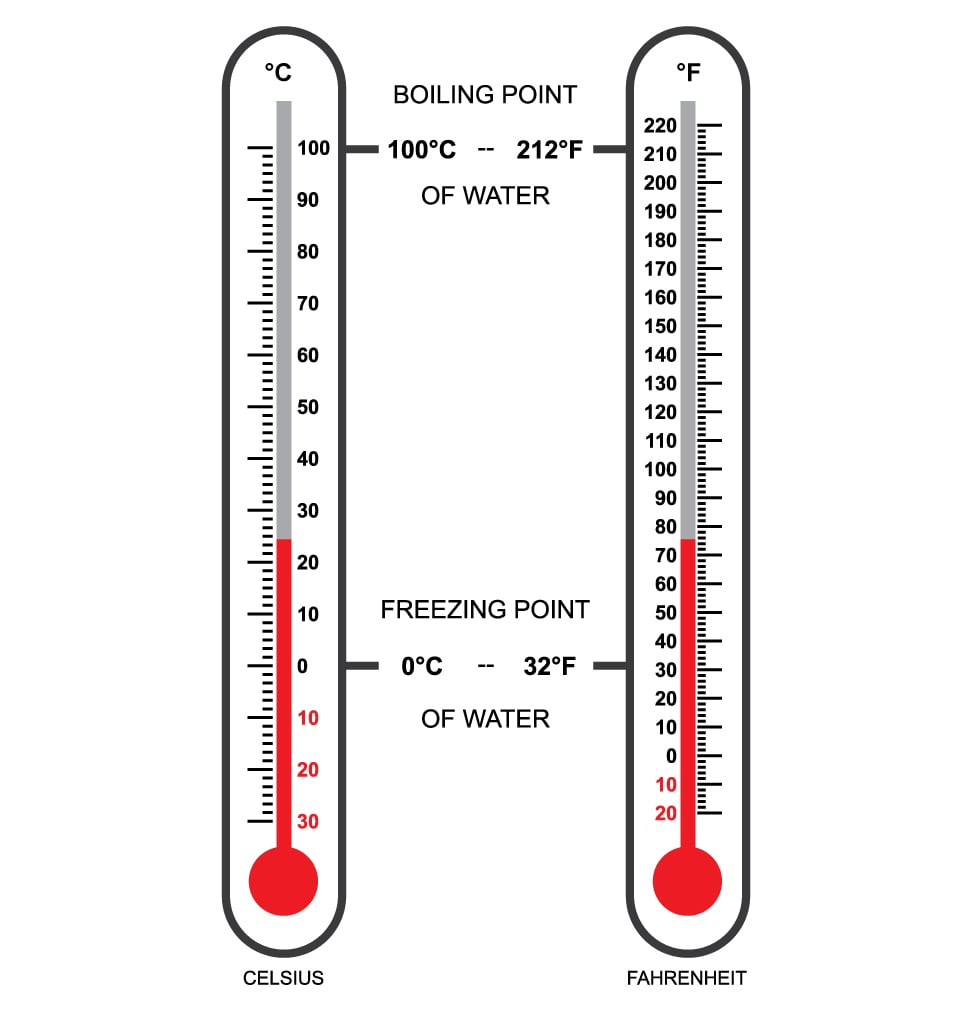# Temperature Converter

## Convert between Celsius, Fahrenheit and Kelvin

### OverviewThe Celsius scale is the most used temperature unit in the world. However, a number of countries, particularly the United States still use the Fahrenheit scale. If you want a quick tool to convert between these two units of temperature, then this tool is for you. This calculator can also convert from and to the Kelvin scale which is the SI unit for temperature.

To use this calculator, select the input type and output type and fill in the input field. Take note that you can specify if you want to convert a single temperature or a range of temperature. To view the results, just click the "calculate" button.

### Equations

$$T_{^0F} = \frac{9}{5}T_{^0C}+32$$

$$T_{^0C} = \frac{5}{9}(T_{^0F}-32)$$

$$T_{K} = T_{^0C} + 273.15$$

### Applications

The temperature unit of Fahrenheit is used to record surface temperature measurements by meteorologists in the United States. Most of the other countries in the world use Celsius. It is important to be able to convert from units of degrees Fahrenheit to degrees Celsius. Kelvin is another unit of temperature that is very handy for many scientific calculations, since it begins at absolute zero (equal to -273.15 0C). This means there is no negative number in the Kelvin scale. Engineers often encounter conversions from Kelvin to Celsius and vice versa.